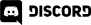# Godot 3 Tutorial–GDScript Programming 101

This video will teach you how to program using the built in scripting language, GDScript.  In addition to learning the basics of GDScript development, this tutorial also walks you through the script editor and teaches you how to debug your code.  It’s a long tutorial at just under 1 hour, but covers a heck of a lot of topics!

## Assets and Code Samples

Example1

```func _ready():
var zeroToNine = Array()
for i in range(10):
zeroToNine.append(i)

print(zeroToNine)
```

Example2

```func _ready():
var a = 1
var b = 2.1
var c = "three"
var d = Vector2(1,0)
var e = String(b) + c

print(e)  # Prints 2.1three
```

Example3

```const  pi = 3.14

var area = radius * pi
print(area) # Prints 6.28
```

Example4

```  var monster = {}
monster.Name = "Orc"
monster.Hp = 42
monster.Alignment = "Chaotic Neutral"
print(monster)
```

Example5

```  var monsters = {
"Orc" : {
"Hp" : 42,
"Alignment" : "Chaotic Neutral"
},
"Elf" : {
"Hp" : 28,
"Alignment" : "Chaotic Good"
}
}
```

Example6

```  if (monsters.Elf.Hp < 5):
print("Elf needs food!")
elif (monsters.Elf.Hp < 10):
print("Elf really wouldn't mind food!")
else:
print("Elf might have an eating problem")
```

Example7

```  var val = 6
match val:
1: print("Value was 1")
2,3,4,5: print("Between 2 and 5")
6:
print("It's 6")
continue
_:print("It's something else")
```

Example8

```extends Node2D

# This is a comment
export var numBoxs = 5
export var dimensions = { Width=200, Height=200 }
var boxLocations = [] # = Array() would be equivalent

# _ready() is one of the callbacks inherited from Node, called after the Node is added to the tree
# Generally this is where you do your setup and configuration
# loop from 0 to numBoxs
for i in range(numBoxs):
#each pass through the loop get a random value for x and y within screen dimensions
var x = rand_range(0, get_viewport().get_visible_rect().size.x)
var y = rand_range(0, get_viewport().get_visible_rect().size.y)
boxLocations.append(Vector2(x,y))

# the _update() function will only be called if you call set_process(true)
set_process(true)

# _update is another function from Node.  Update is called every pass through the game loop
# In this case _update() is actually useless and could be removed.  Done for demo purposes only
func _update(dt):
pass # A function cannot be empty, so you use the token pass

# _draw() is a function inherited from Node2D and can be used to do custom drawing code
func _draw():
# loop through each entry in the boxLocations array, the current value is stored in the variable box
for box in boxLocations:
# draw a red rectangle at each different location stored in the array
var rect = Rect2(box.x,box.y,dimensions.Width,dimensions.Height)
draw_rect(rect,Color(1.0,0.0,0.0))
```